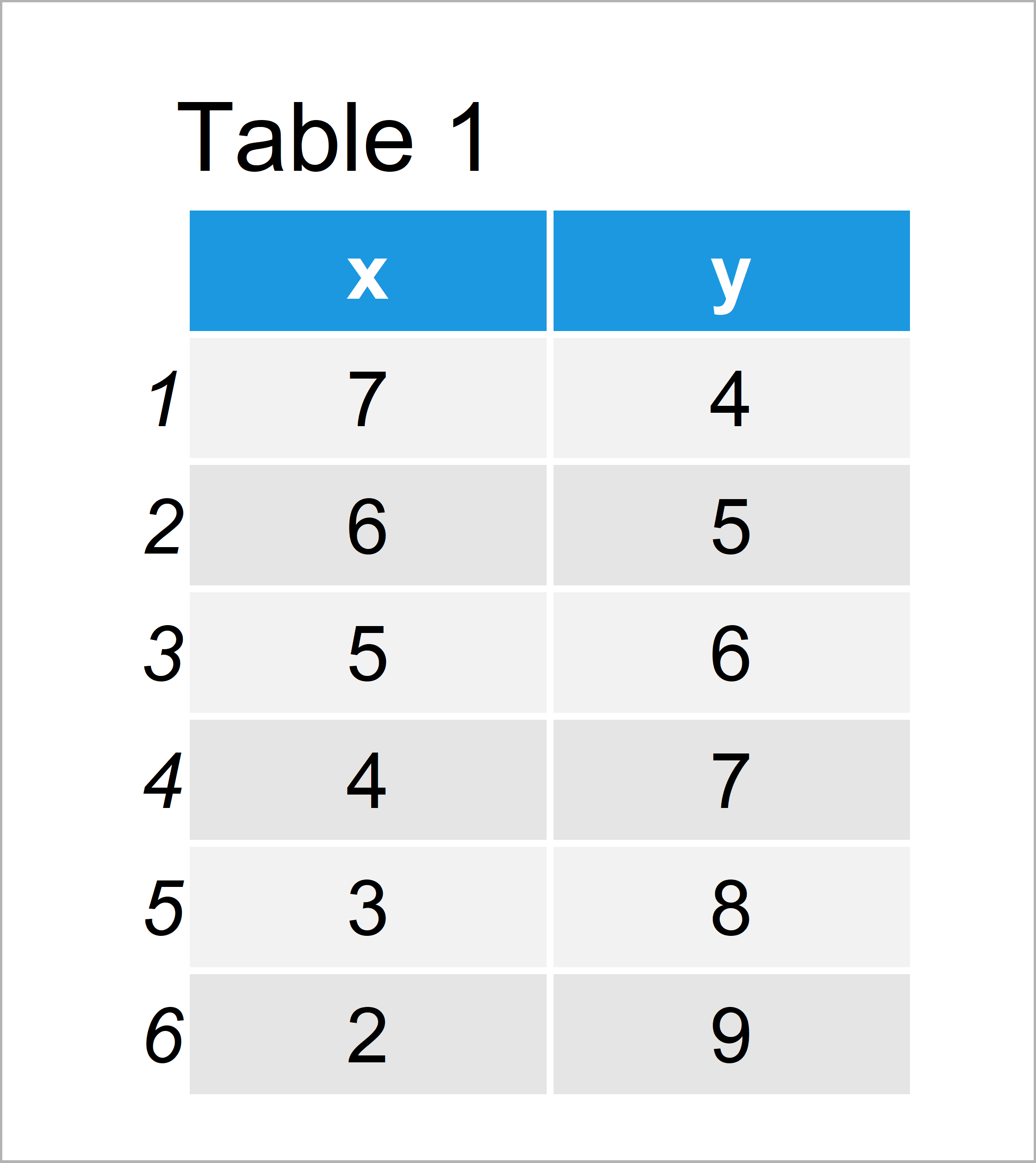# ggplot2 Error in R: “`data` must be a data frame, or other object coercible by `fortify()`, not an integer vector”

This article explains how to deal with the ggplot2 error message “`data` must be a data frame, or other object coercible by `fortify()`, not an integer vector” in R.

The content looks as follows:

Let’s take a look at some R codes in action.

## Exemplifying Data, Packages & Default Graph

We’ll use the following data as basement for this tutorial:

```data <- data.frame(x = 7:2,        # Create example data
y = 4:9)
data                               # Print example data```Table 1 reveals that our example data consists of six data points and two numeric columns.

In this tutorial, we also need to install and load the ggplot2 package.

```install.packages("ggplot2")        # Install ggplot2 package

## Example 1: Reproduce the Error Message – `data` must be a data frame, or other object coercible

This example illustrates how to replicate the error “`data` must be a data frame, or other object coercible by `fortify()`, not an integer vector” in R.

Let’s assume that we want to draw a ggplot2 scatterplot showing the variables x and y of our data:

```ggplot(data\$x, aes(x, y)) +        # False specification in ggplot
geom_point()
# Error: `data` must be a data frame, or other object coercible by `fortify()`, not an integer vector
# Run `rlang::last_error()` to see where the error occurred.```

The previous R code leads to the error message “`data` must be a data frame, or other object coercible by `fortify()`, not an integer vector”.

The reason for that is that we have tried to specify a numeric vector as data argument.

Have a closer look at the first line of our code. We have used the R code “ggplot(data\$x”, i.e. we used the column x as data.

The ggplot2 function, however, needs a data frame as input.

So how can we solve this problem?

## Example 2: Fix the Error Message – `data` must be a data frame, or other object coercible

In Example 2, I’ll explain how to handle the error message “`data` must be a data frame, or other object coercible by `fortify()`, not an integer vector”.

To solve our problem, we have to specify a data frame instead of a vector at the beginning of the ggplot function:

```ggplot(data, aes(x, y)) +          # Properly draw ggplot2 pot
geom_point()```As shown in Figure 1, we have created a scatterplot of our data frame using the previous syntax.

## Video, Further Resources & Summary

Do you need more explanations on the content of this tutorial? Then you might want to watch the following video tutorial of the Statistics Globe YouTube channel. I show the R programming codes of this tutorial in the video:

Please accept YouTube cookies to play this video. By accepting you will be accessing content from YouTube, a service provided by an external third party.If you accept this notice, your choice will be saved and the page will refresh.

Furthermore, you could read the other articles of https://www.statisticsglobe.com/.

To summarize: You have learned in this article how to handle the error “`data` must be a data frame, or other object coercible by `fortify()`, not an integer vector” in the R programming language. In case you have further questions and/or comments, tell me about it in the comments section. Furthermore, please subscribe to my email newsletter in order to get regular updates on new tutorials.

Subscribe to the Statistics Globe Newsletter